﻿ ア メ リ カ   緬 甸   .網     Search_2_Processes_with_OR

Search . 2 processes with OR      Last updated on 2014/2558108, a full moon day;

{TRUE}

Public Logic hijklmno( )Dim file_number   As IntegerDim time_stamp   As IntegerDim x   As IntegerDim y   As Integer

' comment: assign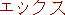x,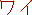y variables' constraint values here; /* Sqrt 2 design only */Dim array (1 To n -1) As Integer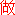DoWhile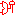TRUEx < min (file_number, time_stamp)

If array(x) = variable Then

file_number =x

Else

array(x) ≠ variablex =x + 2

End If

Loop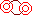{FALSE}ORDoWhileTRUEy < min (file_number, time_stamp)

If array(y) = variable Then

time_stamp =y

Else

array(y) ≠ variabley =y + 2

End If

Loop{FALSE}

hijklmno = min (file_number, time_stamp)

End Logic

{FALSE}

For duo-binary bit developers only: test and develop the above logic for searching ... ;  Add distribution with 6 parameters fuzzy set here;

_ IFF search engine (56366295 IE), ア メ リ カ   緬 甸   .網     this DOMAIN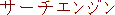;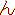for multi dimension, for multi layer, for multi OS, for multi platform, ... , artificial intelligence (meta)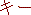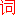keyword ... ;

Develop above Sqrt 2 design 's algorithm to be with Sqrt 3, because dual binary bit testing can be done as of 2014/2558; Also see: Monbusho level knowledge enhancement (idea ♯ 155; Reinventing numerological dimensional numbers) ... ;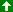Up